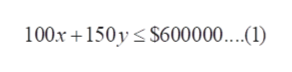# National Business Machines manufactures x model A fax machines and y model B fax machines. Each model A costs $100 to make, and each model B costs$150. The profits are $45 for each model A and$35 for each model B fax machine. If the total number of fax machines demanded per month does not exceed 2500 and the company has earmarked no more than $600,000/month for manufacturing costs, how many units of each model should National make each month to maximize its monthly profit?(x,y)=what is the optimal profit? Question Asked Oct 4, 2019 68 views National Business Machines manufactures x model A fax machines and y model B fax machines. Each model A costs$100 to make, and each model B costs $150. The profits are$45 for each model A and $35 for each model B fax machine. If the total number of fax machines demanded per month does not exceed 2500 and the company has earmarked no more than$600,000/month for manufacturing costs, how many units of each model should National make each month to maximize its monthly profit?

(x,y)=

what is the optimal profit?

check_circle

Step 1

Refer to the question.

There are x model A fax machines and y model B fax machines and each model A costs $100 to make, and each model B costs$150 whereas the company has earmarked no more than $600,000/month for manufacturing costs that is,help_outlineImage Transcriptionclose100x150y 600000...1) fullscreen Step 2 Similarly, the profits are$45 for each model A and \$35 for each model B fax machine. If the total number of fax machines demanded per month does not exceed 2500 so the constraint formed as,

Step 3

Then plot the graph as by finding the intercept and b...

### Want to see the full answer?

See Solution

#### Want to see this answer and more?

Solutions are written by subject experts who are available 24/7. Questions are typically answered within 1 hour.*

See Solution
*Response times may vary by subject and question.
Tagged in

### Linear Programming